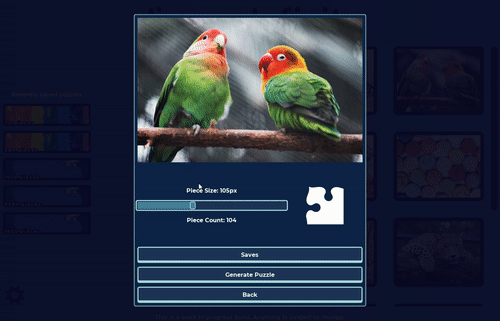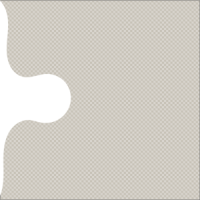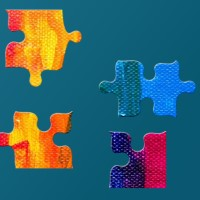Intro

Hi! I’m going to show you how I’m generating randomized puzzle pieces at runtime in my Jigsaw puzzle game (title still pending ;)). I’m using Godot engine, mono version (3.4 as of this post) with C#.

This is my first devlog so it might be a bit rough. Let’s just get to it.

General processes outline

Currently, I’m only doing grid type puzzles with rectangle as a base (and it will probably stay that way). Shapes are applied using a set of masks. I’m heavily leveraging Godot Image class in the process. Each puzzle piece is a separate object with it’s own texture and some additional data. This is how it looks ingame:Full code for puzzle piece generating class - gist.

General algorithm looks more or less like this:

• Calculate puzzle piece amount in X and Y dimensions. Check how many full pieces fit on each axis, cut out an actual puzzle from the middle out
• Generate puzzle pieces side data
• Generate pieces by taking parts of the image and applying masks to it according to the side data lookup
• Add pieces to the scene

1) Calculating actual puzzle size

This one is fairly simple. I take full image size and divide width and height by the piece size (piece size is chosen before puzzle generation). Then I check how many full pieces will fit and calculate actual puzzle size from it. Next I take the full image, and cut out the actual size from the middle out, leaving a part of the edge out.

int puzzleWidth = fullPuzzleTexture.GetWidth();
int puzzleHeight = fullPuzzleTexture.GetHeight();
// 1. Check how many pieces fit and get adjusted width and height
int pieceXCount = puzzleWidth / PieceSize;
int pieceYCount = puzzleHeight / PieceSize;
int adjustedWidth = pieceXCount * pieceSize;
int adjustedHeight = pieceYCount * pieceSize;
// 2. Cut off new size from the full image from middle out
int startingX = (puzzleWidth - adjustedWidth) / 2;
int startingY = (puzzleHeight - adjustedHeight) / 2;

2) Puzzle side data

Here I create a lookup based on piece index (x,y) position, as in top left piece is (0,0), second row left piece would be (0,1) etc.

Starting from top left corner, I go through each column topdown, and then move on to the next one.

SideData is a simple class that holds data for each side of the puzzle in tuples:

Since I’m going top -> down and then left -> right, and the first piece is a corner, I assume that top and left sides can be matched to the adjacent piece (since it was generated already), and bottom and right sides are to be randomized. I randomize two things: 1) Is the side a tab (convex shape) or a blank (concave shape) 2) Which mask will be used for the shape

// Top side of the puzzle
if (puzzlePosition != PuzzlePosition.TOP_LEFT &&
puzzlePosition != PuzzlePosition.TOP &&
puzzlePosition != PuzzlePosition.TOP_RIGHT)
{
Vector2 neighbourPuzzleIndex = puzzleIndex;
neighbourPuzzleIndex.y -= 1;
isTab = !tabsAndBlanks[neighbourPuzzleIndex].Bottom.isTab;

}
// Left side of the puzzle
if (puzzlePosition != PuzzlePosition.TOP_LEFT &&
puzzlePosition != PuzzlePosition.LEFT &&
puzzlePosition != PuzzlePosition.BOT_LEFT)
{
Vector2 neighbourPuzzleIndex = puzzleIndex;
neighbourPuzzleIndex.x -= 1;
isTab = !tabsAndBlanks[neighbourPuzzleIndex].Right.isTab;

}
// Right side of the puzzle
if (puzzlePosition != PuzzlePosition.TOP_RIGHT &&
puzzlePosition != PuzzlePosition.RIGHT &&
puzzlePosition != PuzzlePosition.BOT_RIGHT)
{
int randomKey = (int)GD.RandRange(0, availableMasksKeys.Length - 0.001);

}
// Bottom side of the puzzle
if (puzzlePosition != PuzzlePosition.BOT_LEFT &&
puzzlePosition != PuzzlePosition.BOTTOM &&
puzzlePosition != PuzzlePosition.BOT_RIGHT)
{
int randomKey = (int)GD.RandRange(0, availableMasksKeys.Length - 0.001);

}

Tab/blank randomization is skewed towards 2/2 layout (~80%), since I thought it looked the most natural, with less pieces having 3/1 or 4/0 tab/blank ratio. This is applied after the above code.

float twoTabFactor = 0.8f;
if (result.Left.isTab && result.Top.isTab)
{
if (GD.Randf() < twoTabFactor)
{ result.Bottom.isTab = false;
result.Right.isTab = false;
}
}
// similiar for other combinations of top/left isTab

Side data is serialized and saved to file after it’s generated so the puzzle can be reloaded later with the same layout.

3) Generating puzzle pieces

Now that side data lookup is available, I can generate the actual images for the pieces. This is how a mask and final pieces look:I go through all the pieces again (same order as before, it does not matter here though).

First we just cut out square piece without tabs or blanks:

Then blanks are applied:

{
Vector2 point = Vector2.Zero;

image.Lock();
// 1. Get starting point - middle of side being masked
// 2. Move starting point to align with top left corner of the maskRect
switch (side)
{
case PuzzlePosition.RIGHT:
point.x = image.GetWidth();
point.y = 0;
break;
// ... similiar cases for all sides
// 3. Apply the mask by iterating over the pixels
for (int x = (int)point.x; x < point.x + maskRect.Size.x; x += 1)
{
for (int y = (int)point.y; y < point.y + maskRect.Size.y; y += 1)
{
Color imageColor = image.GetPixel(x, y);
if (color.a > 0.1f)
{
imageColor.a = 1 - color.a;
image.SetPixel(x, y, imageColor);
}
}
}

image.Unlock();

And lastly, tabs are applied:

private Image AddTabsToImage(Image image, Rect2 rect, PuzzleSideData puzzleSideData)
{

// 1. Create new image with target size
Vector2 targetSize = image.GetSize() + topLeft * 2;
Image targetImage = new Image();
targetImage.Create((int)targetSize.x, (int)targetSize.y, false, image.GetFormat());

// 2. Set pixels for base image (starting from top+left resize vector position)
targetImage.BlitRect(image, image.GetUsedRect(), topLeft);

// 3. Get pixels for the tabs from the full puzzle
// 4. Blit mask with image on each side if needed
puzzleSideData.Top.isTab)
{

targetImage.BlitRect(
}
// Similiar for other sides
}

// Copy pixels if alpha != 0
{
image.Lock();

for (int x = 0; x < image.GetWidth(); x += 1)
{
for (int y = 0; y < image.GetHeight(); y += 1)
{

{
image.SetPixel(x, y, new Color(0, 0, 0, 0));
}
{
Color imageColor = image.GetPixel(x, y);
image.SetPixel(x, y, imageColor);
}
}
}
image.Unlock();